0517-86996066
18915186518

#### 联系我们0517-86996066

要：

1) 水箱法。检定前, 用水平仪检查主水箱底座及有机玻璃管支架是否水平。安装待检液位计并调整安装精度, 进行正、反行程检定, 进行逐点控制并读数, 使检定点均匀分布在被检液位计整个测量范围内, 直至液位升降到测量上下限。在示值检定过程的同时, 调整电信号示值, 使之与其检定值一一对应。

2) 比较法。比较法是利用高一级别的长度计量仪器去检定和校准液位计。高一级别的长度计量仪器通常选用高精度激光测距仪、双频激光干涉仪、光栅尺和磁栅尺等一系列高精度仪器。将被检液位计拆卸之后, 运输到高一级别的检定单位 (省计量院、国家计量院) 对该液位计进行标定, 期间可能由于运输、安装等不确定因素对被检液位计本身和其检定结果造成一定的影响。

### 1 浮子式液位计原位校准系统研究

#### 1.1 浮子式液位计测量原理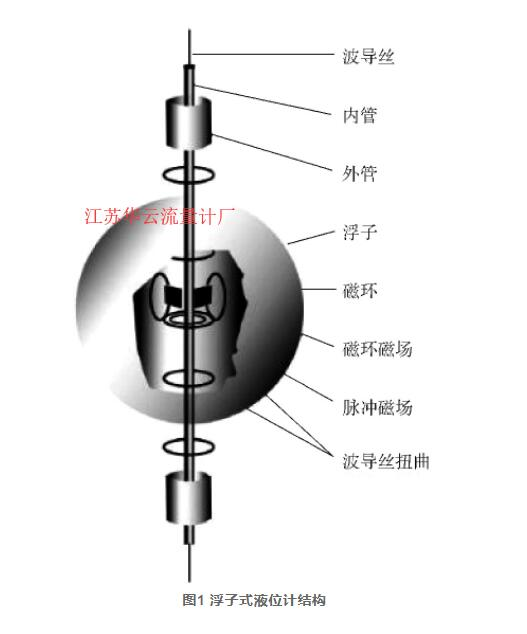#### 1.2 系统整体设计

1) 系统精度设计。本文针对量程为0～6 m、精度为±1 mm的液位计进行校准。根据计量学理论, 标准装置的精度应该高出被检设备的0.3～1个数量级, 故校准装置精度应不大于±0.3 mm, 长度计量仪器精度应不大于±0.1 mm。

2) 系统功能设计。由于系统对浮子式液位计进行现场校准, 且浮子式液位计多在油库油罐等环境使用, 故所用仪器和装置应具有便携、安装方便、操作简单等特点和防爆等功能。根据现场测试环境, 系统采用提升装置托举浮子模拟浮子在液面的运动。

#### 1.3 系统硬件设计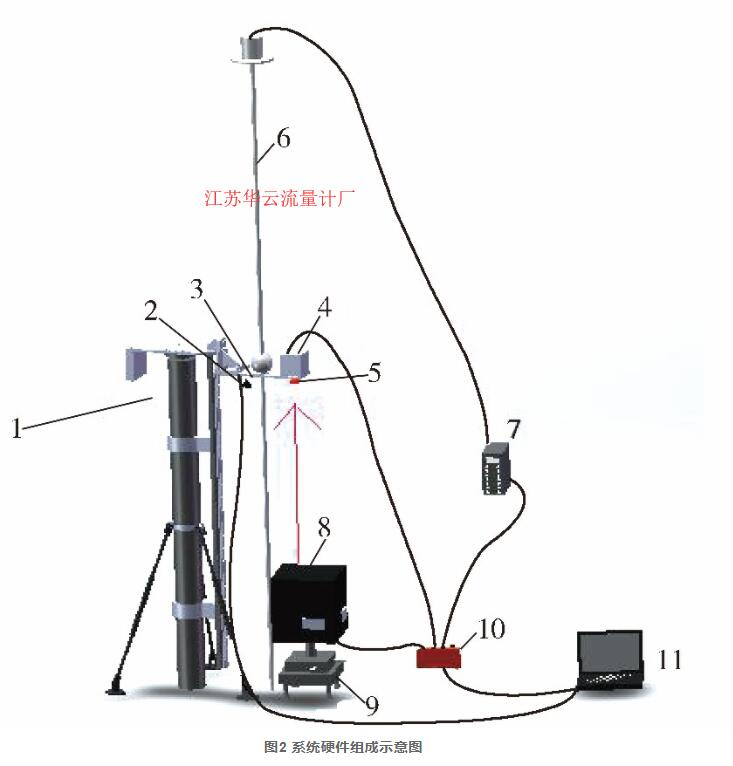1—提升装置;2—摄像机;3—顶板;4—电子倾斜仪 5—反射棱镜;6—浮子式液位计;7—液位计显示器 8—精密测距仪 (内置电子倾斜仪) ;9—可平移基座 10—TLINK控制器;11—PC机

### 2 系统关键技术

#### 2.1 导杆垂直度测量

$\theta =\mathrm{arccos}\frac{6\phantom{\rule{0.25em}{0ex}}000-0.3}{6\phantom{\rule{0.25em}{0ex}}000}\approx 0.57°.\text{ }\text{ }\text{ }\text{ }\text{ }\text{ }\text{ }\text{ }\text{ }\left(1\right)$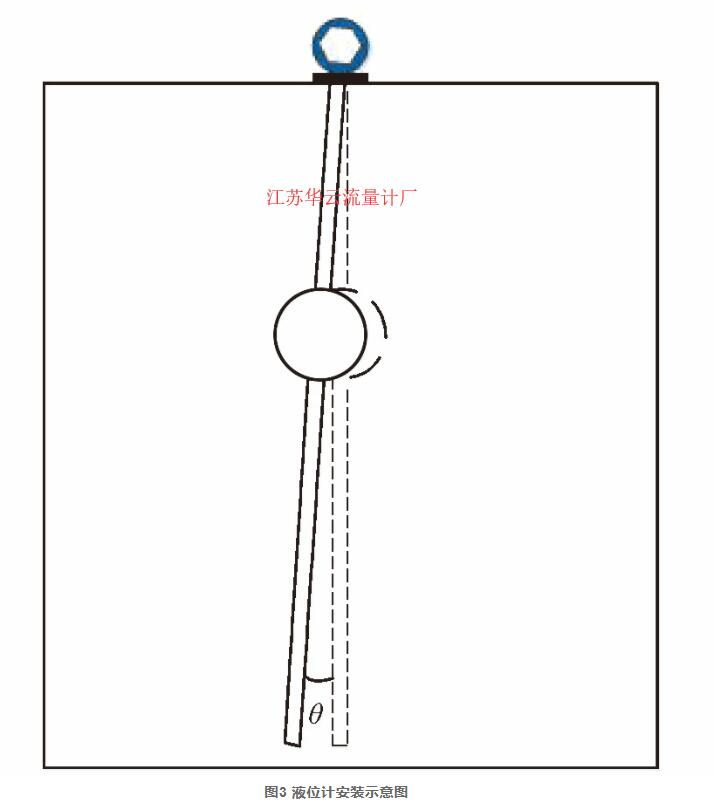$Η{}_{Ζ}=\left(Η{}_{ΖL}+Η{}_{ΖR}\right)/2.\text{ }\text{ }\text{ }\text{ }\text{ }\text{ }\text{ }\text{ }\text{ }\left(2\right)$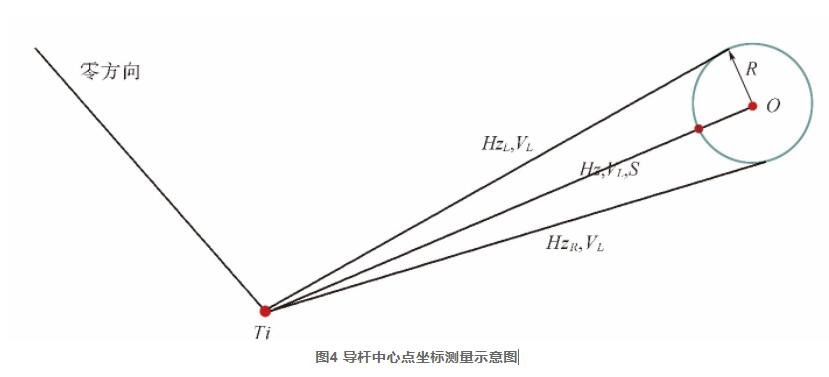$\left\{\begin{array}{l}x{}_{i}=\left(S\text{s}\text{i}\text{n}V{}_{L}+R\right)\mathrm{cos}\phantom{\rule{0.25em}{0ex}}Η{}_{Ζ},\\ y{}_{i}=\left(S\text{s}\text{i}\text{n}\phantom{\rule{0.25em}{0ex}}V{}_{L}+R\right)\mathrm{sin}\phantom{\rule{0.25em}{0ex}}Η{}_{Ζ},\\ z{}_{i}=S\text{c}\text{o}\text{s}\phantom{\rule{0.25em}{0ex}}V{}_{L}.\end{array}\text{ }\text{ }\text{ }\text{ }\text{ }\text{ }\text{ }\text{ }\text{ }\left(3\right)$

#### 2.2 精密测距仪数据内插与修正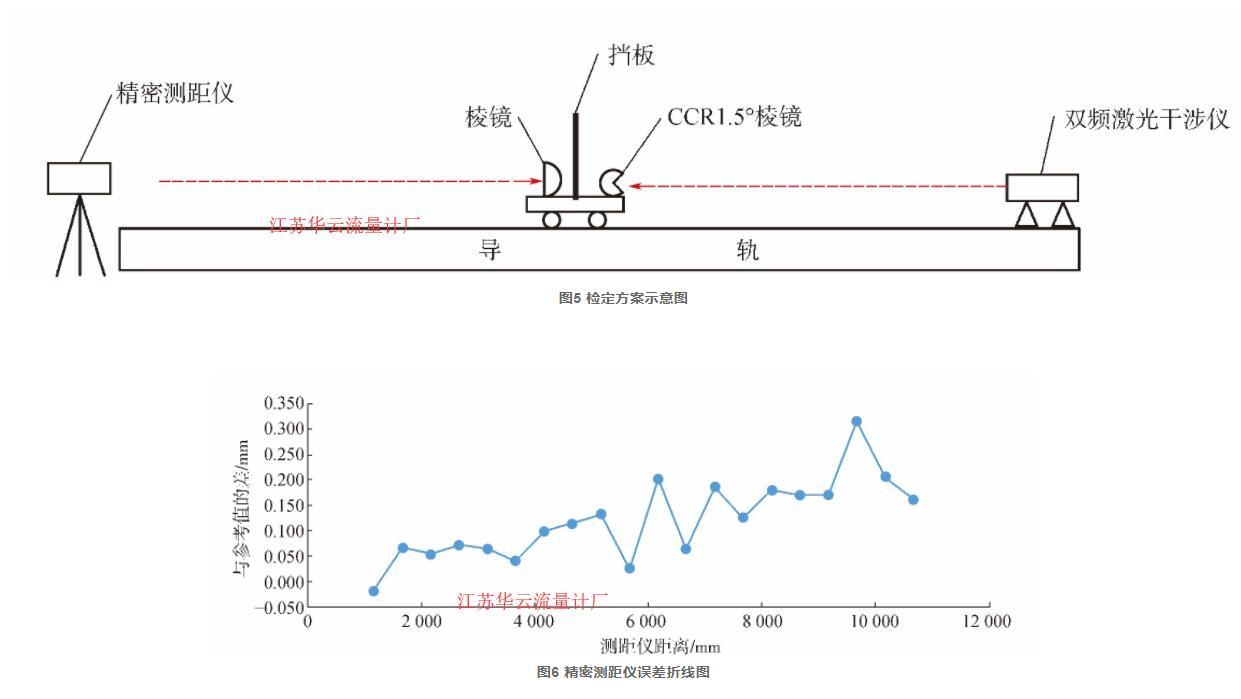$y=0.016\phantom{\rule{0.25em}{0ex}}17x+0.02.\text{ }\text{ }\text{ }\text{ }\text{ }\text{ }\text{ }\text{ }\text{ }\left(4\right)$

#### 2.3 系统倾斜改正

1) 测距轴线倾斜改正。浮子移动距离应归算至铅垂线上, 但测量时测距轴线与铅垂线存在夹角, 故对测距值进行倾斜改正。如图8所示。

$\text{Δ}=L-Η=L-L\text{c}\text{o}\text{s}\phantom{\rule{0.25em}{0ex}}\alpha .\text{ }\text{ }\text{ }\text{ }\text{ }\text{ }\text{ }\text{ }\text{ }\left(5\right)$

2) 顶部装置倾斜改正。由于提升装置的加工误差、伸缩杆不垂直等原因导致顶板发生倾斜, 引起测距值的偏差, 如图9所示。

$\gamma =D×\mathrm{sin}\phantom{\rule{0.25em}{0ex}}\beta .\text{ }\text{ }\text{ }\text{ }\text{ }\text{ }\text{ }\text{ }\text{ }\left(6\right)$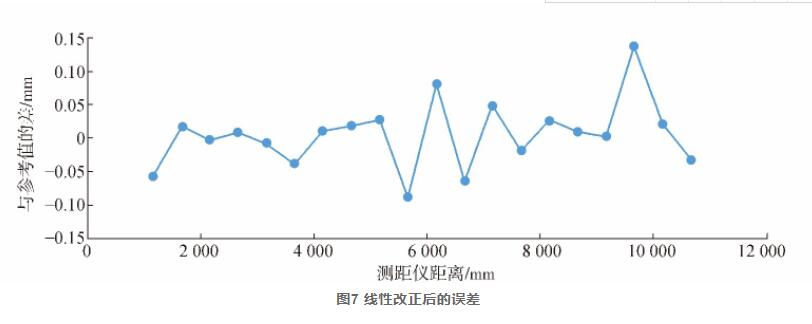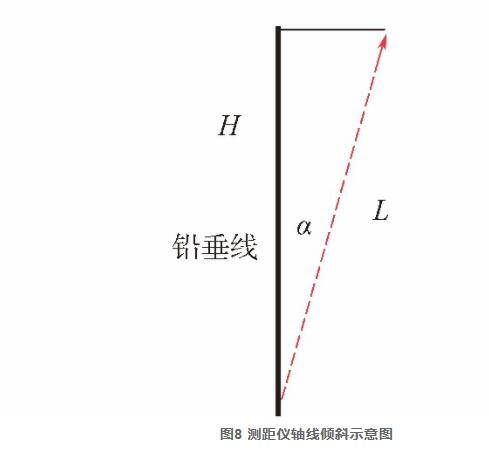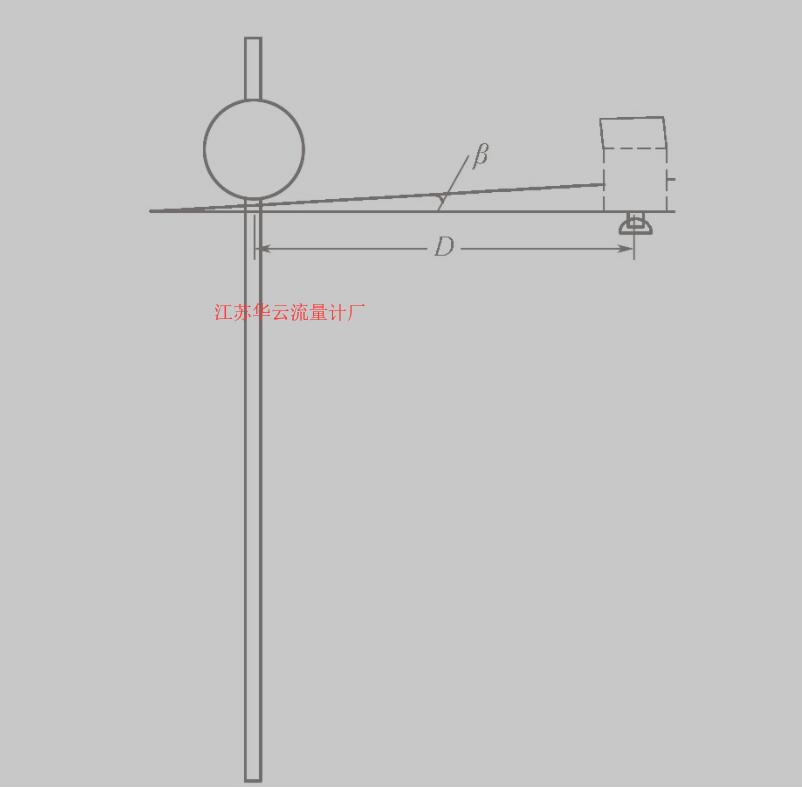3) 实验验证及数据分析。由于实验室内条件的限制, 本文对一个标称精度为±1 mm、量程为3 m的液位计进行了实验测试。实验室内环境温度为20 ℃, 导杆的垂直度为0.346°, 实验数据如表1所示。

1) 系统误差的判别。用不同公式计算标准差比较法判断测量数据是否存在系统误差。

mm

 液位计 精密测距仪 液位值 参考值 差值 0.00 241.37 0.00 0.00 0.00 311.33 556.65 311.33 315.28 -3.95 517.00 762.70 205.67 206.05 -0.38 972.33 1 217.14 455.33 454.44 0.89 1 337.00 1 583.70 364.67 366.56 -1.89 1 871.67 2 117.69 534.67 533.99 0.68 2 369.67 2 615.99 498.00 498.30 -0.30 2 865.67 3 110.62 496.00 494.64 1.36 2 365.67 2 611.35 -500.00 -499.27 -0.73 1 866.67 2 111.52 -499.00 -499.83 0.83 1342.00 1 586.65 -524.67 -524.87 0.20 981.33 1 227.32 -360.67 -359.33 -1.34 584.67 830.09 -396.67 -397.23 0.56 317.00 563.57 -267.67 -266.52 -1.15 24.67 271.04 -292.33 -292.54 0.20 RMS=1.40

$\sigma {}_{1}=\sqrt{\frac{\sum _{i=1}^{n}v{}_{i}^{2}}{n-1}}.\text{ }\text{ }\text{ }\text{ }\text{ }\text{ }\text{ }\text{ }\text{ }\left(7\right)$

$\sigma {}_{2}=1.253\frac{\sum _{i=1}^{n}|v{}_{i}|}{\sqrt{n\left(n-1\right)}}.\text{ }\text{ }\text{ }\text{ }\text{ }\text{ }\text{ }\text{ }\text{ }\left(8\right)$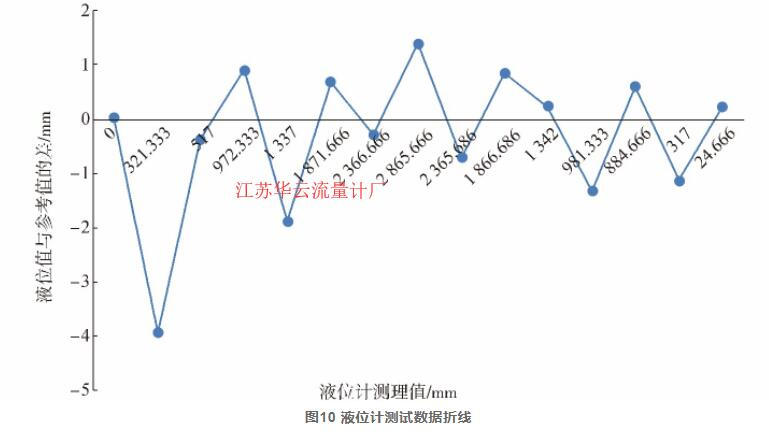$\begin{array}{l}\text{令}\frac{\sigma {}_{2}}{\sigma {}_{1}}=1+\mu .\text{ }\text{ }\text{ }\text{ }\text{ }\text{ }\text{ }\text{ }\text{ }\left(9\right)\\ \text{若}|\mu |\ge \frac{2}{\sqrt{n-1}}.\text{ }\text{ }\text{ }\text{ }\text{ }\text{ }\text{ }\text{ }\text{ }\left(10\right)\end{array}$

 σ1 σ2 σ2σ1$\frac{\sigma {}_{2}}{\sigma {}_{1}}$ |μ|$|\mu |$ 分析结果 1.31 1.20 0.91 0.31 因为|μ|<2n−1√$|\mu |<\frac{2}{\sqrt{n-1}}$, 未发现系统误差

2) 粗差的判别。在测量中, 粗差主要是由测量条件异常变化产生的误差, 一般以异常值或孤值形式表现出来。本文利用3σ (莱以特准则) 对粗差进行判断和剔除。表3是对实验数据的粗差分析结果。

 ΔMAX σ 3σ 分析结果 -3.97 1.31 3.93 因为|ΔMAX|>3σ , 存在粗差, 剔除

3) 精度分析。通过实验数据分析得到, 粗差产生的原因是液位计读数出现跳动引起的, 将第一次实验数据的粗差剔除后重新计算均方根误差为RMS=0.94 mm, 液位计测试精度满足其标称精度。通过对精度为±1 mm的浮子式液位计进行原位校准, 验证了校准装置的稳定性和可靠性。